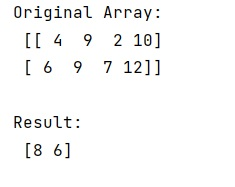# numpy.ptp() Method with Example

Learn about the numpy.ptp() method with its usage, syntax, and example.
Submitted by Pranit Sharma, on March 21, 2023

## numpy.ptp() Method

The numpy.ptp() method is used to find out the range of values along an axis. Here, the values are defined as the difference between the maximum values and the minimum values along an axis. This method always preserves the data type of the array. It means that the output for a signed integer with n bits is also a signed integer with n bits.

## Syntax

```numpy.ptp(a, axis=None, out=None, keepdims=<no value>)
```

## Parameter(s)

• a: input array values
• axis: axis along which this function will find the range of values
• out: an alternative output array to place the result.
• keepdims: A bool type. It is an optional parameter. If we set ii to True, the axes which are reduced are left in the result as dimensions with size one.

## Return Value

It returns the range of the given array.

## Example of numpy.ptp() method in Python

```# Import numpy
import numpy as np

# Creating a numpy array
arr =  np.array([[4, 9, 2, 10],[6, 9, 7, 12]])

# Display original array
print("Original Array:\n",arr,"\n")

# Finding a range of values
res = np.ptp(arr,axis=1)

# Display result
print("Result:\n",res,"\n")
```

### OutputReference: numpy.ptp()